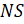THE EARTH AS A SPHERE

Mathematics SSS 3 First Term

WEEK 8

THE EARTH AS A SPHERE

Performance Objectives

Student should be able to:

1. Describe the earth as a sphere and identify the lines of longitude, latitude, equator, north pole and south pole, small circle and great circle.
2. Calculate the distance along the great circle.
3. Calculate radius of parallel of latitudes.
4. Calculate distance along the parallel of latitudes

Content

Various photographs of the earth taken from space has revealed that the earth is spherical in shape and has a radius varying between 6300 km and 6400 km. The figure below shows a model of the earth which consists of the following: The North and South poles, The polar axis NSView More